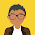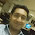## Monday, April 12, 2010

### how to prove it - intro exercises

Ex-1 (a)
Using the formula
\$2^{15} - 1\$ = \$2^{3*5} -1\$
a = 5, b = 3
\$2^b -1\$ = \$2^3 - 1\$ = 7

and

\$1 + 2^b + 2^{2b} + ... + 2^{(a-1)b}\$ = \$1 + 2^3 + 2^{2*3} + 2^{3*3} + 2^{4*3}\$ = 4681

Ex-1 (b)
\$2^{32767} - 1\$ = \$2^{7*4681} - 1\$
b = 7, so
x = \$2^7 - 1\$ = 127

Ex-2

 n \$3^n - 1\$ \$3^n - 2^n\$ 1 2 1 2 8 5 3 26 19 4 80 65 5 242 211 6 728 665 7 2186 2059 8 6560 6305 9 19682 19171 10 59048 58025

2 conjectures are:
\$3^n - 2^n\$ is prime for prime n
\$3^n - 1\$ is never prime except for n = 1

Ex-3(a)
2*3*5*7 + 1 = 211

Ex-3(b)
2*3*5*7*11 + 1 = 2311

Ex-4
x = (n+1)! + 2 = (5+1)! + 2 = 722
5 consecutive non-prime numbers are x, x + 1, x + 2, x + 3, x + 4 = 722,723,724,725,726

Ex-5
Use: If \$2^n - 1\$ is prime \$\Rightarrow\$ \$2^{(n-1)}(2^n - 1)\$ is perfect number
\$2^5 - 1 = 31\$ is prime \$\Rightarrow\$ \$2^{(5-1)}(2^5 - 1)\$ = 496 is perfect number
\$2^7 - 1 = 127\$ is prime \$\Rightarrow\$ \$2^{(7-1)}(2^7 - 1)\$ = 8128 is perfect number

Ex-6 There are no more triplet primes other than 3,5,7 where adjacent numbers in the list differ by 2.

Proof: I'll prove it by contradiction. Let say p, p+2, p+4 (p > 3)be such a triplet prime list.

Since one of the 3 consecutive numbers has to be divisible by 3, so
one out of p, p+1 or p+2 is divisible by 3. Since p and p+2 are primes by assumption so p+1 is divisible by 3. But then p+4 = (p+1)+3 and hence p+4 should be divisible by 3 and this is a contradiction.

#### 13 comments:

1.Just noticed - in your solution to Ex 6, 57 is not a prime - it's 19 x 3.

I was doing the same exercises, and came across this - http://en.wikipedia.org/wiki/Prime_triplet

2.thanks hrish. Now that I think about it again, I think another list of such triplet primes is not possible and I've added a quick proof for same.

just curious, what was your answer?

3.Himanshu, Initially I was under the impression that such a triplet might exist, and it might do so within the first 100 or 150 +ve integers. I actually went through the numbers and noticed the pattern in the wikipedia article I mention. A google search found it for me - so I did not actually prove it myself. Wish I had done that first :)

4.don't worry, there will be plenty of opportunities :)

5.BTW in Ex-3(b), the answer is not 111 because 111 is not a prime; It is divisible by 3.

6.thanks @Niyaz, yes I missed 3 and 7. For the theorem to work I have to include all the primes from 2 to 11. Corrected that now.

7.Hmm... the answer is correct, but this method is still not guaranteed to give you a prime number. (If it did, then it would be easy to find a larger prime number than the current largest prime number right? http://en.wikipedia.org/wiki/Largest_known_prime_number )

My point was that 111 is not a prime number. 111 = 3 * 37, which gives us 37 (or 3) as our new prime number which the question asked us to find.

If you look at the proof of theorem 3, you can see that there are two cases in it. First when p1.p2.p3....pn + 1 is a prime and the second case where it is not a prime. I think Ex-3(a) wants us to use case 1 while Ex-3(b) wants us to use case 2.

8.@Niyaz: No, the method is guaranteed to give you a prime.
2*3*5*....p_n + 1 will always be a prime number(however, ensure that you are taking all the primes smaller than and equal to p_n, you can't miss any). I guess, finding the largest prime is limited by the computational resources and not by theory. As theorem is all about proving that there are infinitely many primes.

9.E6 = 13# + 1 = 30031 = 59 x 509 is the first composite Euclid number, demonstrating that not all Euclid numbers are prime.

http://en.wikipedia.org/wiki/Euclid_number

10.Thanks for correcting.. Yes, Agree, I was wrong.

There exist infinite primes *does not* imply all euclid numbers are prime(which looked like true due to the way theorem was proved).

11.Stumbling across this in 2013 while working through the introduction; I think the above discussion should be mandatory reading after attempting the exercise!

12.In fact, 3^n-2^n is a multiple of 5 whenever n is even. (Can be proved by induction.)

13.The conjecture that '3^n-2^n is a prime number when n is prime' is not valid. This can be seen for n=7 where 3^7-2^7=2059=29*71.